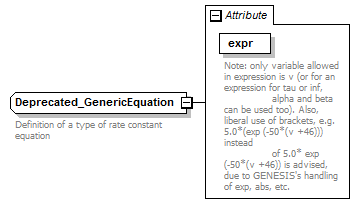# Deprecated_GenericEquation

 `Definition of a type of rate constant equation`

## Model## Attributes

QName Type Fixed Default Use Inheritable Annotation
expr xs:string required
 ```Note: only variable allowed in expression is v (or for an expression for tau or inf, alpha and beta can be used too). Also, liberal use of brackets, e.g. 5.0*(exp (-50*(v +46))) instead of 5.0* exp (-50*(v +46)) is advised, due to GENESIS's handling of exp, abs, etc.```

## Used By

 Elements Deprecated_RateConstVoltConcDep/generic, Deprecated_RateConstVoltConcDep/generic_equation_hh, Deprecated_RateConstantEqnChoice/generic, Deprecated_RateConstantEqnChoice/generic_equation_hh

## Source

 ``` Definition of a type of rate constant equation Note: only variable allowed in expression is v (or for an expression for tau or inf, alpha and beta can be used too). Also, liberal use of brackets, e.g. 5.0*(exp (-50*(v +46))) instead of 5.0* exp (-50*(v +46)) is advised, due to GENESIS's handling of exp, abs, etc. ```